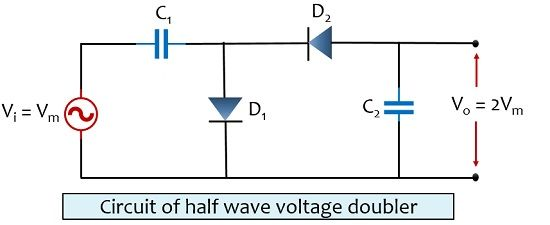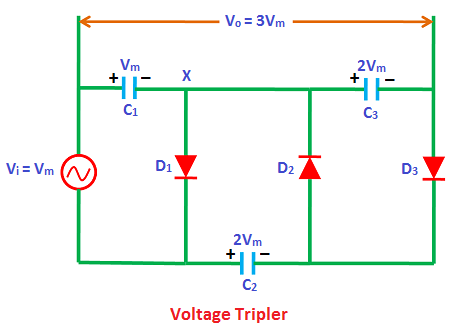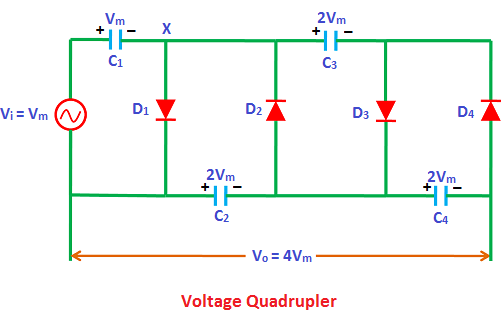### Voltage Doubler

Voltage Doubler:

The output voltage is double the input voltage of ac input.In above circuit capacitor c1 is charge through diode  and can store maximum voltage of Vm ie peak value of input AC voltage. In negative half cycle  voltage across capacitor C2 is two times the input  peak voltage voltage.

Voltage Trippler:

In this circuit output voltage is three times the maximum value of input voltage.